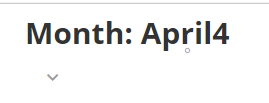# I am looking for html language to hide metrics within a beast mode summary number calculation

Hey all,

I'm looking for html language to use in a summary number beast mode calculation to hide certain metrics so only the month portion is visible.  I'm looking to hide everything from view in the summary number after the (`Post Month`) portion of the first 2 lines.  Is this possible?  Here's the beast mode I'm using (apologize for the length):

CONCAT('<div style="line-height:1.5">',
'<div style="font-size: 12px"><b>Month: ',  Monthname(`Post Month`),
'<div style="font-size: 12px"><b>TY Room Revenue: \$',
Case when (SUm(Case when Year(`Post Month`) = YEAR(CURDATE()) and `Rollup3` = 'Room Revenue' and `Type` = 'Actuals' and  `Account Type` = 'Income' then (`Amount`) end)) >=1000000 then

Concat(  Round( ( SUm(Case when Year(`Post Month`) = YEAR(CURDATE()) and `Rollup3` = 'Room Revenue' and `Type` = 'Actuals' and  `Account Type` = 'Income' then (`Amount`) end))/1000000,2),'M')

when ((SUm(Case when Year(`Post Month`) = YEAR(CURDATE()) and `Rollup3` = 'Room Revenue' and `Type` = 'Actuals' and  `Account Type` = 'Income' then (`Amount`) end)) >=1000 and

(SUm(Case when Year(`Post Month`) = YEAR(CURDATE()) and `Rollup3` = 'Room Revenue' and `Type` = 'Actuals' and  `Account Type` = 'Income' then (`Amount`) end)) <1000000) then

Concat(  Round( ( SUm(Case when Year(`Post Month`) = YEAR(CURDATE()) and `Rollup3` = 'Room Revenue' and `Type` = 'Actuals' and  `Account Type` = 'Income' then (`Amount`) end))/1000,2),'K')

else SUm(Case when Year(`Post Month`) = YEAR(CURDATE()) and `Rollup3` = 'Room Revenue' and `Type` = 'Actuals' and  `Account Type` = 'Income' then (`Amount`) end)
end ,

'<div style="font-size: 12px"><b>Budget Room Revenue: \$',
Case when (SUm(Case when Year(`Post Month`) = YEAR(CURDATE()) and `Rollup3` = 'Room Revenue' and `Type` = 'Budget' and  `Account Type` = 'Income' then (`Amount`) end)) >=1000000 then

Concat(  Round( ( SUm(Case when Year(`Post Month`) = YEAR(CURDATE()) and `Rollup3` = 'Room Revenue' and `Type` = 'Budget' and  `Account Type` = 'Income' then (`Amount`) end))/1000000,2),'M')

when ((SUm(Case when Year(`Post Month`) = YEAR(CURDATE()) and `Rollup3` = 'Room Revenue' and `Type` = 'Budget' and  `Account Type` = 'Income' then (`Amount`) end)) >=1000 and

(SUm(Case when Year(`Post Month`) = YEAR(CURDATE()) and `Rollup3` = 'Room Revenue' and `Type` = 'Budget' and  `Account Type` = 'Income' then (`Amount`) end)) <1000000) then

Concat(  Round( ( SUm(Case when Year(`Post Month`) = YEAR(CURDATE()) and `Rollup3` = 'Room Revenue' and `Type` = 'Budget' and  `Account Type` = 'Income' then (`Amount`) end))/1000,2),'K')

else SUm(Case when Year(`Post Month`) = YEAR(CURDATE()) and `Rollup3` = 'Room Revenue' and `Type` = 'Budget' and  `Account Type` = 'Income' then (`Amount`) end)
end ,

'<div style="font-size: 12px"><b>LY Room Revenue: \$',
Case when (SUm(Case when Year(`Post Month`) = YEAR(CURDATE())-1 and `Rollup3` = 'Room Revenue' and `Type` = 'Actuals' and  `Account Type` = 'Income' then (`Amount`) end)) >=1000000 then

Concat(  Round( ( SUm(Case when Year(`Post Month`) = YEAR(CURDATE()) -1 and `Rollup3` = 'Room Revenue' and `Type` = 'Actuals' and  `Account Type` = 'Income' then (`Amount`) end))/1000000,2),'M')

when ((SUm(Case when Year(`Post Month`) = YEAR(CURDATE())-1 and `Rollup3` = 'Room Revenue' and `Type` = 'Actuals' and  `Account Type` = 'Income' then (`Amount`) end)) >=1000 and

(SUm(Case when Year(`Post Month`) = YEAR(CURDATE())-1 and `Rollup3` = 'Room Revenue' and `Type` = 'Actuals' and  `Account Type` = 'Income' then (`Amount`) end)) <1000000) then

Concat(  Round( ( SUm(Case when Year(`Post Month`) = YEAR(CURDATE()) -1 and `Rollup3` = 'Room Revenue' and `Type` = 'Actuals' and  `Account Type` = 'Income' then (`Amount`) end))/1000,2),'K')

else SUm(Case when Year(`Post Month`) = YEAR(CURDATE())-1 and `Rollup3` = 'Room Revenue' and `Type` = 'Actuals' and  `Account Type` = 'Income' then (`Amount`) end)
end )

• CONCAT('<div style="line-height:1.5">',
'<div style="font-size: 12px"><b>Month: ',  Monthname(max(`Post Month`)))

______________________________________________________________________________________________
“There is a superhero in all of us, we just need the courage to put on the cape.” -Superman
______________________________________________________________________________________________

• You mean that you only want to see the Post Month?

CONCAT('<div style="line-height:1.5">',
'<div style="font-size: 12px"><b>Month: ',  Monthname(`Post Month`))

Should do it.  If you are looking for something else, please elaborate or provide an example of what you are looking for.

______________________________________________________________________________________________
“There is a superhero in all of us, we just need the courage to put on the cape.” -Superman
______________________________________________________________________________________________
• @ST_-Superman-_

I am looking to show only the Post Month as a Summary Number using a beast mode.  I already tried what you provided earlier, but the Dimension is not showing within the Field & Function options under Summary Number, and I'm not sure why (I did click on 'Save Calculation to Dataset').  The original contractor stated I would need to use HTML language to hide the additonal metrics while keeping them there so only the Post Month label will appear because of the setup of Summary Number within the system.  Unfortunately, that contract is expended so they cannot research the right language for me to use unless I add more hours to the contract.  So, here I am seeing if the community may have a work around.

• Try creating a new summary number:

CONCAT(MAX(MONTH(`Post Month`)))

______________________________________________________________________________________________
“There is a superhero in all of us, we just need the courage to put on the cape.” -Superman
______________________________________________________________________________________________
• @ST_-Superman-_

Using the CONCAT function with the Max Month gives me the number of 4 (4 properties showing 4 months of data).  I added the original language to add the `Post Month` and it shortened the beast mode to this:

CONCAT('<div style="line-height:1.5">',
'<div style="font-size: 12px"><b>Month: ',  Monthname(`Post Month`),
MAX(MONTH(`Post Month`)))

Here is how it looks:I would like to be able to hide the number 4 that appears.  I can't add just the `Post Month` as that is not a summary of anything.  So, I need to keep the summary component, yet hide it from view on the card.

• @ST_-Superman-_

YOU ARE AMAZING!!!  THANK YOU!!!